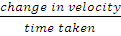### Equation of uniformly accelerated motion

Physics SSS2 First Term

Sub Theme: Interaction of matter, space and time

WEEK 4

Equation of uniformly accelerated motion

Performance Objectives

Students should be able to;

1. Deduce the three equations of motion from a (v – t) graph with initial velocity and constant acceleration.
2. Explain the terms used in the equations of motion

Analysis of Rectilinear Motion

Content

Supposing a body moving at an initial velocity later attains a final velocity in time.

Its acceleration is given as:

a =Change in velocity = v − u

a = v−ut– – – – – -(1)

By making v subject of the formula,

We have v = u + at – – – – – – (2)

Recall that speed = distance/time

View More